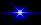# Shotgun Microphone

Created "May 23, 2012"

```
Years ago I built a project very Simular to this.
I am not going to duplicate it again, But I wish to share some details
to help others that might want to build one.

My origional unit used 1/4 Inch Thin wall Aluminum Tubing.
And I Double Coated each tube with Contact Cement.
This aided Both in Assembly as well as adding a Soft Surface on Each tube
to Repel sounds coming from the Sides.

However Tubes can be 1/4 to 1/2 inch diameter in either Plastic or Metal.
Seems most people use 3/8" diameter tubing.

It is ESSENTIAL to Insulate around the Funnel and Microphone
to prevent sounds from entering on the Back Plane.
Possibly you could put a small Metal or Plastic Can, with Foam in it, Enclosing the Mic and Funnel.

Based on Tube Lengths for Most of these "Plans Found on the Internet".
This results in a Considerable "Emphased BASS" Frequency Response.
So this would Not be good for listening to BIRDS.

Since the Ends of these tubes are enclosed with a funnel and Microphone,
The Frequencies for the CLOSED Ends may be More Correct.

Here are the Frequencies for tubes from 1 Inch to 36 Inches.

LENGTH   FREQUENCY For OPEN ENDED TUBES     FREQUENCY For CLOSED ENDED TUBES

1" =       6,766 Hz.                             3.383 Hz.
2" =       3,383 Hz.                             1,691 Hz.
3" =       2,255 Hz.                             1.127 Hz.
4" =       1,691 Hz.                               845 Hz.
5" =       1.363 Hz.                               681 Hz.
6" =       1,128 Hz.                               564 Hz.
7" =         967 Hz.                               484 Hz.
8" =         846 Hz.                               423 Hz.
9" =         752 Hz.                               376 Hz.
10" =         677 Hz.                               339 Hz.
11" =         615 Hz.                               308 Hz.
12" =         564 Hz.                               282 Hz.
13" =         520 Hz.                               260 Hz.
14" =         483 Hz.                               242 Hz.
15" =         451 Hz.                               226 Hz.
16" =         423 Hz.                               212 Hz.
17" =         398 Hz.                               199 Hz.
18" =         376 Hz.                               188 Hz.
19" =         356 Hz.                               178 Hz.
20" =         338 Hz.                               169 Hz.
21" =         322 Hz.                               161 Hz.
22" =         307 Hz.                               154 Hz.
23" =         294 Hz.                               147 Hz.
24" =         282 Hz.                               141 Hz.
25" =         271 Hz.                               136 Hz.
26" =         260 Hz.                               130 Hz.
27" =         251 Hz.                               126 Hz.
28" =         241 Hz.                               121 Hz.
29" =         233 Hz.                               117 Hz.
30" =         226 Hz.                               113 Hz.
31" =         218 Hz.                               109 Hz.
32" =         211 Hz.                               106 Hz.
33" =         205 Hz.                               103 Hz.
34" =         199 Hz.                               100 Hz
35" =         193 Hz.                                97 Hz.
36" =         188 Hz.                                94 Hz.

Due to the Funnel (Added Space) used to help reflect these sounds into the Microphone,
The actual frequencies may be somewhat lower than as shown above.

A More Suitable Approach would be Tube Lengths, Cut for 1/4 Octave Frequencies.
This would result in a "MORE LINEAR" Frequency Response

Frequency for         Frequency for      Tube Length   Tube Length
Open Ended Tubes      Closed End Tubes     in Cm.        in Inches.

16000.0 Hz.                   8000.0 Hz. =            .53               0.209
13454.4 Hz.                   6727.2 Hz. =            .64               0.252
11313.8 Hz.                   5656.9 Hz. =            .76               0.299
9513.6 Hz.                   4756.8 Hz. =            .90               0.354
8000.0 Hz.                   4000.0 Hz. =           1.07               0.421
6727.2 Hz.                   3363.6 Hz. =           1.28               0.504
5656.8 Hz.                   2828.4 Hz. =           1.52               0.598
4756.8 Hz.                   2378.4 Hz. =           1.81               0.713
4000.0 Hz.                   2000.0 Hz. =           2.15               0.846
3363.6 Hz.                   1681.8 Hz. =           2.55               1.00
2828.4 Hz.                   1414.2 Hz. =           3.04               1.20
2378.4 Hz.                   1189.2 Hz. =           3.61               1.42
2000.0 Hz.                   1000.0 Hz. =           4.29               1.69
1681.8 Hz.                    840.9 Hz. =           5.11               2.01
1414.2 Hz.                    707.1 Hz. =           6.08               2.29
1189.2 Hz.                    594.6 Hz. =           7.22               2.84
1000.0 Hz.                    500.0 Hz. =           8.60               3.39
840.8 Hz.                    420.4 Hz. =          10.22               4.02
707.2 Hz.                    353.6 Hz. =          12.15               4.78
594.6 Hz.                    297.3 Hz. =          14.45               5.57
500.0 Hz.                    250.0 Hz. =          17.19               6.77
420.4 Hz.                    210.2 Hz. =          20.44               8.05
353.6 Hz.                    176.8 Hz. =          24.30               9.57
297.4 Hz.                    148.7 Hz. =          28.89              11.37
250.0 Hz.                    125.0 Hz. =          34.37              13.53
210.2 Hz.                    105.1 Hz. =          40.88              16.09
176.8 Hz.                     88.4 Hz. =          48.60              19.13
148.6 Hz.                     74.3 Hz. =          57.82              22.76
125.0 Hz.                     62.5 Hz. =          68.74              27.06
105.2 Hz.                     52.6 Hz. =          81.68              32.16
88.4 Hz.                     44.2 Hz. =          97.20              38.27
74.4 Hz.                     37.2 Hz. =         115.49              45.47
62.5 Hz.                     31.25 Hz. =        137.48              54.13

Due to the Funnel (Adding Space) used to help reflect these sounds into the Microphone,
The actual frequencies may be somewhat lower than as shown above."Back to My HOME Page"CLICK HERE, a Basic Diagram